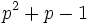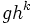# Semidirect product of cyclic group of prime-square order and cyclic group of prime order

View other such prime-parametrized groups

## Definition

Let$p$ be an odd prime. This group is, up to isomorphism, the only non-abelian group of order$p^3$ and exponent$p^2$.

Note that for$p = 2$, these descriptions work, and yield dihedral group:D8 -- however, that group has a very different qualitative behavior from the odd$p$ case.

### Notation

This group is sometimes denoted as$M_3(p)$ or as$M_{p^3}$.

### As a Sylow subgroup of a holomorph

This group is isomorphic to the$p$-Sylow subgroup of the holomorph of the cyclic group of order$p^2$.

### As a presentation

In non-abelian language, it is a group given by the presentation:$\langle g,h \mid g^{p^2} = h^p = e, gh = hg^{p+1} \rangle$

Here$g$ is the generator of a cyclic normal subgroup of order$p^2$ and$h$ is the generator of the complement of order$p$ acting on it.

### As a semidirect product

Given a prime$p$, this group is defined as the semidirect product of the cyclic group$\Z/p^2\Z$ with the cyclic group$\Z/p\Z$, where$a \in \Z/p\Z$ acts on$x \in \Z/p^2\Z$ as follows:$a.x = (p+1)^ax = (ap + 1)x$

More explicitly, it is the group of ordered pairs$(u,v)$ with$u \in \Z/p^2\Z$ and$v \in \Z/p\Z$ with multiplication given by:$(u,v) * (s,t) = (u + (vp+1)s, v + t)$

## Related groups

For any prime$p$, there are two non-Abelian groups of order$p^3$: this one and the group of upper triangular unipotent matrices over the prime field.

## Arithmetic functions

Compare and contrast arithmetic function values with other groups of prime-cube order at Groups of prime-cube order#Arithmetic functions

For some of these, the function values are different when$p = 2$ and/or when$p = 3$. These are clearly indicated below.

### Arithmetic functions taking values between 0 and 3

Function Value Explanation
prime-base logarithm of order 3 the order is$p^3$
prime-base logarithm of exponent 2 the exponent is$p^2$.
nilpotency class 2
derived length 2
Frattini length 2
minimum size of generating set 2
subgroup rank 2
rank as p-group 2
normal rank as p-group 2
characteristic rank as p-group 2 the subgroup comprising the identity element and the elements of order$p$ is a characteristic elementary abelian subgroup of order$p^2$.

### Arithmetic functions of a counting nature

Function Value Explanation
number of conjugacy classes$\! p^2 + p - 1$$p$ elements in the center, and each other conjugacy class has size$p$
number of subgroups$\! 2p + 4$ when$p \ne 2$,$10$ when$p = 2$
number of normal subgroups$\! p + 4$
number of conjugacy classes of subgroups$\! p + 5$ for$p \ne 2$,$8$ for$p = 2$

## Subgroups

The subgroups are as follows:

1. The trivial subgroup. (1)
2. The center, which is the subgroup$\langle g^p \rangle$, or the multiples of$p$ in the cyclic group. It is also the commutator subgroup, the Frattini subgroup and the socle. (That all these are the same indicates that this group is an extraspecial group). Isomorphic to group of prime order. (1)
3. Subgroups of order$p$ generated by conjugates of$h$. They form a single conjugacy class of size$p$. ($p$)
4. The subgroup of order$p^2$ generated by$g^p$ and$h$: in other words, the multiples of$p$ in the cyclic normal subgroup, and the element of order$p$ acting on it. This is a fully invariant subgroup. Isomorphic to elementary abelian group of prime-square order. (1)
5. Cyclic normal subgroups of order$p^2$, generated by elements of the form$gh^k$. All these are automorphic to the cyclic subgroup$\langle g \rangle$, though each one is normal, so no two of them are conjugate. Isomorphic to cyclic group of prime-square order. ($p$)
6. The whole group. (1)

Types (1), (2), (4) and (6) are characteristic, while type (5) is normal not not characteristic. Type (3) comprises automorph-conjugate subgroups that are not normal.

## Endomorphisms

### Inner automorphisms

The inner automorphisms preserve the normal subgroup$\Z/p^2\Z$, and they act via multiplication of every element in it by$ap+1$ for$a$ in$\Z/p\Z$.

### Outer automorphisms

These automorphisms permute the subgroups of order$p^2$. Here are some examples of outer automorphisms:$(1,0) \mapsto (x_0,a_0), (0,1) \mapsto (0,1)$

where$x_0$ is an invertible element modulo$p^2$.

Note that no automorphism can take$(0,a)$ to$(0,a')$ for distinct$a$ and$a'$. The retract comprising$(0,a)$s is thus a quasicharacteristic retract. Since the automorphism group of the kernel is an Abelian group, we in fact conclude that the retract is a relatively rigid subgroup.